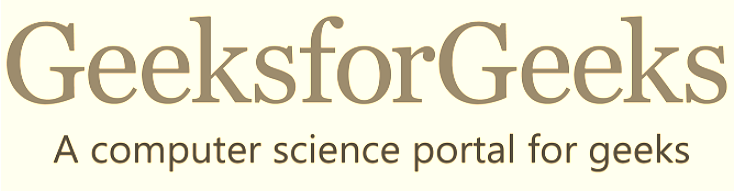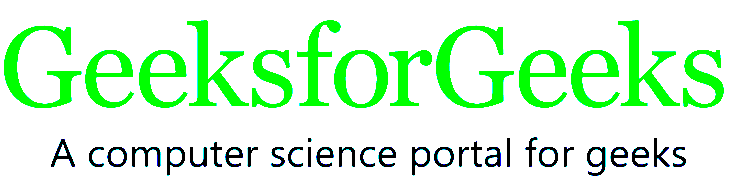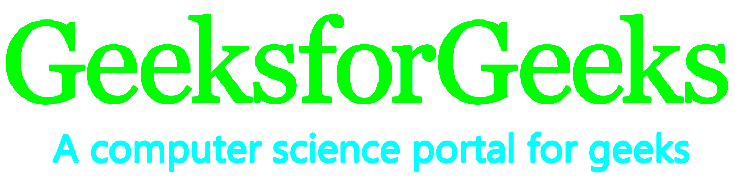# CSS | filter Property

The filter property is used to set the visual effect of an element. This property is mostly used in image content.

Syntax:

```filter: none|blur()|brightness()|contrast()|drop-shadow()|
grayscale()|hue-rotate()|invert()|opacity()|saturate()|sepia()|
url();```

Note: More then one filter can be added to the HTML element.
Example:

```img {
filter: brightness(20%) blur(20px);
}```

This example applying two filter function to the image element in a webpage.

Filter function:

• none: It is the default value and it does not apply any effect.

Example:

 ` ` `<``html``> ` `     `  `<``head``> ` `    ``<``title``>CSS filter property ` `    ``<``style``> ` `        ``img { ` `            ``filter: none; ` `        ``} ` `    `` ` ` ` `<``body``> ` `    ``<``div``> ` `        ``<``img` `src` `=  ` `"https://media.geeksforgeeks.org/wp-content/uploads/geeksforgeeks-25.png"`  `        ``alt``=``"Geeks image"` `> ` `    `` ` ` ` ` `  `                     `

Output:• brightness(): It sets the brightness of the element. If the brightness is 0% then it is completely black and if the brightness is 100% then it is same as original. The values greater than 100% result is brighter elements.

Example:

 ` ` `<``html``> ` `     `  `<``head``> ` `    ``<``title``>CSS filter property ` `    ``<``style``> ` `        ``img { ` `            ``filter: brightness(10%); ` `        ``} ` `    `` ` ` ` `<``body``> ` `    ``<``div``> ` `        ``<``img` `src` `=  ` `"https://media.geeksforgeeks.org/wp-content/uploads/geeksforgeeks-25.png"`  `        ``alt``=``"Geeks image"` `> ` `    `` ` ` ` ` `  `                     `

Output:• grayscale(): It converts the element colors into black and white. The grayscale 0% indicates the original element and 100% indicate completely grayscale element.

Example:

 ` ` `<``html``> ` `     `  `<``head``> ` `    ``<``title``>CSS filter property ` `    ``<``style``> ` `        ``img { ` `            ``filter: grayscale(70%); ` `        ``} ` `    `` ` ` ` `<``body``> ` `    ``<``div``> ` `        ``<``img` `src` `=  ` `"https://media.geeksforgeeks.org/wp-content/uploads/geeksforgeeks-25.png"`  `        ``alt``=``"Geeks image"` `> ` `    `` ` ` ` ` `  `                     `

Output:• sepia(): It converts the image into sepia image where 0% represents the original image and 100% represents completely sepia.

Example:

 ` ` `<``html``> ` `     `  `<``head``> ` `    ``<``title``>CSS filter property ` `    ``<``style``> ` `        ``img { ` `            ``filter: sepia(50); ` `        ``} ` `    `` ` ` ` `<``body``> ` `    ``<``div``> ` `        ``<``img` `src` `=  ` `"https://media.geeksforgeeks.org/wp-content/uploads/geeksforgeeks-25.png"`  `        ``alt``=``"Geeks image"` `> ` `    `` ` ` ` ` `  `                     `

Output:• contrast() It helps to adjust the contrast of the element. The 0% contrast indicate complete black element and 100% contrast indicate original element.

Example:

 ` ` `<``html``> ` `     `  `<``head``> ` `    ``<``title``>CSS filter property ` `    ``<``style``> ` `        ``img { ` `            ``filter: contrast(50); ` `        ``} ` `    `` ` ` ` `<``body``> ` `    ``<``div``> ` `        ``<``img` `src` `=  ` `"https://media.geeksforgeeks.org/wp-content/uploads/geeksforgeeks-25.png"`  `        ``alt``=``"Geeks image"` `> ` `    `` ` ` ` ` `  `                     `

Output:• saturate(): It is used to set saturation of element. The 0% saturate indicate element completely unsaturated and 100% saturate indicate the original image. The value greater than 100% result is super-saturated element.

Example:

 ` ` `<``html``> ` `     `  `<``head``> ` `    ``<``title``>CSS filter property ` `    ``<``style``> ` `        ``img { ` `            ``filter: saturate(50); ` `        ``} ` `    `` ` ` ` `<``body``> ` `    ``<``div``> ` `        ``<``img` `src` `=  ` `"https://media.geeksforgeeks.org/wp-content/uploads/geeksforgeeks-25.png"`  `        ``alt``=``"Geeks image"` `> ` `    `` ` ` ` ` `  `                     `

Output:• blur(): It applies blur effect to the element. If blur value is not specified then it takes 0 as default value.

Example:

 ` ` `<``html``> ` `     `  `<``head``> ` `    ``<``title``>CSS filter property ` `    ``<``style``> ` `        ``img { ` `            ``filter: blur(5px); ` `        ``} ` `    `` ` ` ` `<``body``> ` `    ``<``div``> ` `        ``<``img` `src` `=  ` `"https://media.geeksforgeeks.org/wp-content/uploads/geeksforgeeks-25.png"`  `        ``alt``=``"Geeks image"` `> ` `    `` ` ` ` ` `  `                     `

Output:• opacity(): It sets the opacity effect of the image. The 0% opacity indicate the element is completely transparent and if opacity is 100% then it indicate the original image.

Example:

 ` ` `<``html``> ` `     `  `<``head``> ` `    ``<``title``>CSS filter property ` `    ``<``style``> ` `        ``img { ` `            ``filter: opacity(0.5); ` `        ``} ` `    `` ` ` ` `<``body``> ` `    ``<``div``> ` `        ``<``img` `src` `=  ` `"https://media.geeksforgeeks.org/wp-content/uploads/geeksforgeeks-25.png"`  `        ``alt``=``"Geeks image"` `> ` `    `` ` ` ` ` `  `                     `

Output:• hue-rotate(): It applies a hue rotation to the image. The value defines the number of degrees around the color circle the image samples will be adjusted. The default value is 0 degree and it represents the original image.

Example:

 ` ` `<``html``> ` `     `  `<``head``> ` `    ``<``title``>CSS filter property ` `    ``<``style``> ` `        ``img { ` `            ``filter: hue-rotate(45deg); ` `        ``} ` `    `` ` ` ` `<``body``> ` `    ``<``div``> ` `        ``<``img` `src` `=  ` `"https://media.geeksforgeeks.org/wp-content/uploads/geeksforgeeks-25.png"`  `        ``alt``=``"Geeks image"` `> ` `    `` ` ` ` ` `  `                     `

Output:• invert(): It inverts the element. The default value is 0% and it represents the original image. The 100% makes the image completely inverted.

Example:

 ` ` `<``html``> ` `     `  `<``head``> ` `    ``<``title``>CSS filter property ` `    ``<``style``> ` `        ``img { ` `            ``filter: invert(50); ` `        ``} ` `    `` ` ` ` `<``body``> ` `    ``<``div``> ` `        ``<``img` `src` `=  ` `"https://media.geeksforgeeks.org/wp-content/uploads/geeksforgeeks-25.png"`  `        ``alt``=``"Geeks image"` `> ` `    `` ` ` ` ` `  `                     `

Output:Example:

 ` ` `<``html``> ` `     `  `<``head``> ` `    ``<``title``>CSS filter property ` `    ``<``style``> ` `        ``img { ` `            ``filter: drop-shadow(16px 18px 15px rgba(255, 0, 0, 0.5)); ` `        ``} ` `    `` ` ` ` `<``body``> ` `    ``<``div``> ` `        ``<``img` `src` `=  ` `"https://media.geeksforgeeks.org/wp-content/uploads/geeksforgeeks-25.png"`  `        ``alt``=``"Geeks image"` `> ` `    `` ` ` ` ` `  `                     `

Output:• initial: It sets the filter property to its default value.

Example:

 ` ` `<``html``> ` `     `  `<``head``> ` `    ``<``title``>CSS filter property ` `    ``<``style``> ` `        ``img { ` `            ``filter: initial(); ` `        ``} ` `    `` ` ` ` `<``body``> ` `    ``<``div``> ` `        ``<``img` `src` `=  ` `"https://media.geeksforgeeks.org/wp-content/uploads/geeksforgeeks-25.png"`  `        ``alt``=``"Geeks image"` `> ` `    `` ` ` ` ` `  `                     `

Output:Supported Browsers: The browsers supported by filter property are listed below:

• Google Chrome 53.0, 18.0 -webkit-
• Internet Explorer 13.0
• Firefox 35.0
• Safari 9.1, 6.0 -webkit-
• Opera 40.0, 15.0 -webkit-

My Personal Notes arrow_drop_upCheck out this Author's contributed articles.

If you like GeeksforGeeks and would like to contribute, you can also write an article using contribute.geeksforgeeks.org or mail your article to contribute@geeksforgeeks.org. See your article appearing on the GeeksforGeeks main page and help other Geeks.

Please Improve this article if you find anything incorrect by clicking on the "Improve Article" button below.

Article Tags :

Be the First to upvote.

Please write to us at contribute@geeksforgeeks.org to report any issue with the above content.